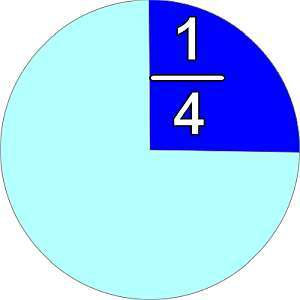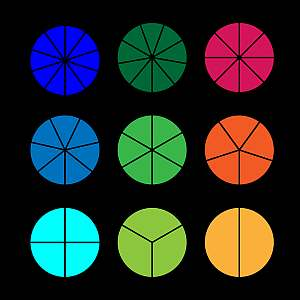# Fraction Calculator

Instructions: Use this fraction calculator to compute any fraction operation or calculation you provide, showing all the steps. Pleas type in the fraction calculation you want to carry out in the form box below.Enter the fraction expression you want to calculator (Ex: 2/3 + 4/5, etc.)

This calculator will allow you adding fractions, multiplying fractions, dividing fractions, etc., and any valid fraction operation, showing all the steps. You need to provide a valid expression involving fractions. It could be something simple as '1/2 + 1/3', or something more complex like '(1/3+1/4)(1/5+1/6)'.

Once you provide a valid expression involving fraction, all you need to do is to click on the "Calculate" button, and you will be provided with all the steps of the calculations.

Fractions algebra involves fraction conversion such as the use of common denominator, and the use of basic arithmetic rules. All in all, the process of the calculation can be laborious, although it can be done systematically, without much of a problem.Adding fractions is one of the most crucial and basic skills you will use when calculating fraction operations. Usually, you need to start with finding a common denominator, but often times you will use the following formula for fraction addition:

$\displaystyle \frac{a}{b} + \frac{c}{d} = \displaystyle \frac{ad + cb}{bd}$

## What are the steps for adding fractions?

• Step 1: Identify the numerator and denominator for the first and second fraction
• Step 2: Assume that a and b are the numerator and denominator of the first fraction, and c and d are the numerator and denominator of the second fraction
• Step 3: Use the addition formula: The resulting fraction has ad + cb as the numerator, and bd as the denominator

Subtracting fractions is just derived by the sum of fractions: To subtract two fractions, you just multiply the second one by -1, and the add it to the first.

## How to multiply fractions?

The second corner stone to conduct general fraction calculations is multiplying fractions. In this case, there is no need to find a common denominator, you will just multiply the numerators and denominators together:

$\displaystyle \frac{a}{b} \times \frac{c}{d} = \displaystyle \frac{ac}{bd}$

## What are the steps for multiplying fractions?

• Step 1: Identify the numerator and denominator for the first and second fraction
• Step 2: Assume that a and b are the numerator and denominator of the first fraction, and c and d are the numerator and denominator of the second fraction
• Step 3: Use the addition formula: The resulting fraction has ad + cb as the numerator, and bd as the denominator

Similar to what happened with addition and subtraction, dividing fractions is just derived from the multiplication of fractions: To divide two fractions, you just multiply first one by the inverse fraction of the second one (the inverse fraction is obtained by swapping the numerator by the denominator in the fraction).

## Why would care about computing fractions?

Fractions are one of the corner stones of algebra and of any general algebraic expression to calculate. Fractions are simple operands, but which can be compounded into more complicated terms using operations like sum, multiplication, etc., and then using functions we can construct even more advanced expressions.

The center of all algebraic calculator starts with the power of basic numbers of fractions.### Example: Calculating the sum of fractions

Calculate the following: $$\frac{1}{3} + \frac{5}{4} - \frac{5}{6}$$

Solution:

We need to calculate and simplify the following expression: $$\displaystyle \frac{1}{3}+\frac{5}{4}-\frac{5}{6}$$.

The following calculation is obtained:

$$\displaystyle \frac{1}{3}+\frac{5}{4}-\frac{5}{6}$$
Amplifying in order to get the common denominator 12
$$= \,\,$$
$$\displaystyle \frac{1}{3}\cdot\frac{4}{4}+\frac{5}{4}\cdot\frac{3}{3}-\frac{5}{6}\cdot\frac{2}{2}$$
Finding a common denominator: 12
$$= \,\,$$
$$\displaystyle \frac{1\cdot 4+5\cdot 3-5\cdot 2}{12}$$
Expanding each term: $$4+5 \times 3-5 \times 2 = 4+15-10$$
$$= \,\,$$
$$\displaystyle \frac{4+15-10}{12}$$
$$= \,\,$$
$$\displaystyle \frac{9}{12}$$
We can factor out 3 for both the numerator and denominator.
$$= \,\,$$
$$\displaystyle \frac{3\cdot 3}{3\cdot 4}$$
Now we cancel 3 out from the numerator and denominator.
$$= \,\,$$
$$\displaystyle \frac{3}{4}$$

which concludes the calculation.

### Example: Another fraction calculation

Calculate $$\left(\frac{2}{3} \times \frac{6}{5} \right)+ \frac{2}{5}$$.

Solution:

We need to calculate and simplify the following expression: $$\displaystyle \left(\frac{2}{3}\cdot\frac{6}{5}\right)+\frac{2}{5}$$.

The following calculation is obtained:

$$\displaystyle \left(\frac{2}{3} \times \frac{6}{5} \right)+ \frac{2}{5}$$
We can multiply the terms in the top and bottom as in $$\displaystyle\frac{ 2}{ 3} \times \frac{ 6}{ 5}= \frac{ 2 \times 6}{ 3 \times 5}$$
$$= \,\,$$
$$\displaystyle \frac{2\cdot 6}{3\cdot 5}+\frac{2}{5}$$
We can factor out the term $$\displaystyle 3$$ in the numerator and denominator in $$\displaystyle \frac{ 2 \times 6}{ 3 \times 5}$$
$$= \,\,$$
$$\displaystyle \frac{2\cdot 2}{5}+\frac{2}{5}$$
After canceling out the common factors
$$= \,\,$$
$$\displaystyle \frac{4}{5}+\frac{2}{5}$$
We use the common denominator: 5
$$= \,\,$$
$$\displaystyle \frac{4+2}{5}$$
$$= \,\,$$
$$\displaystyle \frac{6}{5}$$

which concludes the calculation.

## Other useful fraction calculators

Fraction calculations are crucial in Algebra. Other useful operations include simplifying a fraction by reducing to its lowest terms. Also, you can convert fraction to percentage or fraction to decimal, as those have an intimate connection.

Also, you may be interested in a mixed fraction calculator, depending on your learning setting. In more elementary settings, mixed numbers are treated as important entities, whereas in more advanced settings, mixed numbers are just presented in their fraction notation..